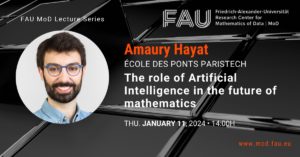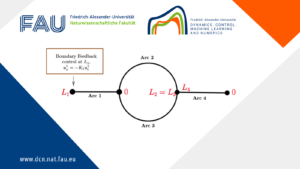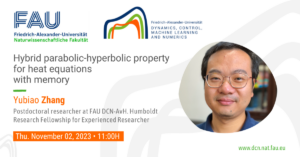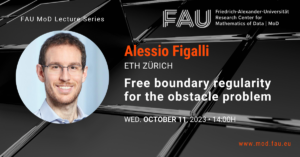# Jean-Daniel Djida

Jean-Daniel Djida
Postdoctoral Researcher

jean-daniel.djida@fau.de
Room 03.364 | Friedrich-Alexander-Universität Erlangen Nürnberg. FAU DCN-AvH Chair for Dynamics, Control, Machine Learning and Numerics – Alexander von Humboldt Professorship.
+49 9131 85-67171

My primary research interest is the analysis of Partial Differential Equations, both from the theoretical and from the numerical point of view, with a particular emphasis on non-local models, control theory, evolution equations, and shape optimisation.

My current research interests cover numerous areas of PDE analysis and control, including: different classes of non-local models such as elliptic, parabolic, and hyperbolic fractional PDE, memory-type equations; numerical analysis for local and non-local PDE with control purposes; controllability properties of PDE and hyperbolic systems arising on continuum models of production and in Machine Learning; analysis and control of collecting behavior models and their micro-macro limit such as mean field equations. Besides, I am working on the development of mathematical and computational tools for the model in Deep learning and fractional PDEs.

PhD Thesis: Some nonlocal operators in porous medium equations: the extension problem and regularity theory (2019)

### My posts#### Analysis of a local-nonlocal polymer chain model

Analysis of a local-nonlocal polymer chain model By Jean-Daniel Djida, Postdoctoral Researcher at FAU DCN-AvH What does the mathematics of ...

### You might like!#### FAU MoD Lecture: The role of Artificial Intelligence in the future of mathematics#### Limits of the stabilization of a networked hyperbolic system with a circle#### Hybrid parabolic-hyperbolic property for heat equations with memory